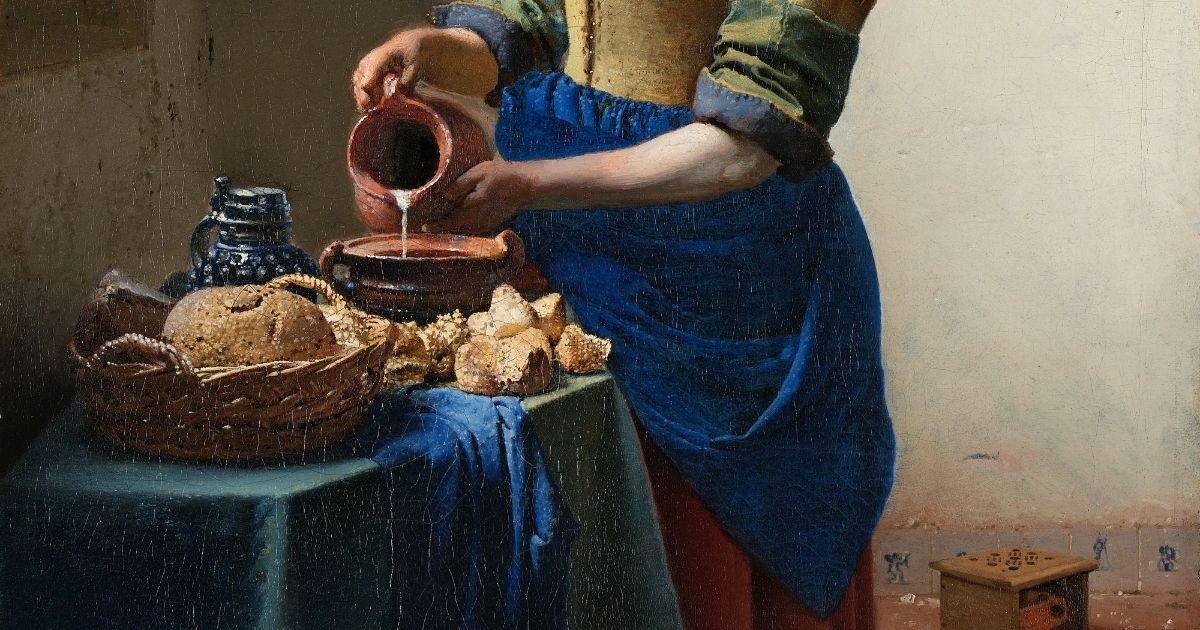Hunter's Woods PH

# Analysis of the Squares - Binomial (Montessori Math)

## Analysis of the squares is an impressionistic introduction to squares and binomials that can lay the groundwork for future higher Maths. Two easy worksheets are included for follow-up work.This is a hundred square.

It has 10 beads on one side and 10 on the other.
10 x 10 = 100

The square is divided by two imaginary lines into 4 parts.

In the first part, there are 6 beads on one side and 6 on the other.
6 x 6 = 36

On the upper right part, there are 6 beads on one side and 4 on the other.
6 x 4 = 24

The part on the lower left has 4 beads on one side and 6 on the other.
4 x 6 = 24

Finally, in the last part, there are 4 beads on one side and 4 on the other.
4 x 4 = 16

Now let’s add the products of the four parts.
36 + 24 + 24 + 16 = 100

The whole [square] is the sum of its parts!

When you’re older, you will learn that you can look at it this way:
10 squared = (6 + 4) squared = (6 + 4) x (6 + 4) = (6 x 6) + (6 x 4) + (4 x 6) + (4 x 4) = 100

Note on the Worksheets

You can reduce the size of the worksheet by zooming out your browser screen. For Windows users, scroll down the mouse wheel while pressing the Ctrl key in your keyboard.

### Worksheet 1: Analysis of the Squares - Binomial

You can get a free printable copy of this worksheet here: Montessori Math Analysis of the Squares Binomial – Worksheet 1 (PDF)

### Worksheet 2: Analysis of the Squares - Binomial

You can get a free printable copy of this worksheet here: Montessori Math Analysis of the Squares Binomial – Worksheet 2 (PDF)

## Trending Quizzes### Dino Quiz! Level 1 Challenge### Greek Mythology Quiz: Can You Identify These Greek Gods and Goddesses?### How Well Do You Know Our Solar System?